# Practice Doc: SNAP 2019 For Analytical & Logical Reasoning Notes - CAT

## CAT: Practice Doc: SNAP 2019 For Analytical & Logical Reasoning Notes - CAT

The document Practice Doc: SNAP 2019 For Analytical & Logical Reasoning Notes - CAT is a part of CAT category.
All you need of CAT at this link: CAT

1. Choose the word that will complete the second pair in the same way as first pair Royal Bengal Tiger: India:: Snow Leopard:
(a) Sri Lanka
(c) UAE
(d) Afghanistan

Ans. (d)
Solution:
There may be two possible answers for this question. Royal Bengal Tiger resides in India, same way Snow Leopard which resides in cold areas is found in Afghanistan as well as in Pakistan.

2. A new species lays exactly 120 eggs out of which 50% are male and 50% are female. The female insect hatch and grow in a span of 20  days to lay eggs by themselves. On 1st May, 2019, an insect laid 120 eggs. How many eggs will be hatched, (approximately) by the end of June 2019?
(a) 216000
(b) 25920000
(c) 432000
(d) None of these
Ans.
(d)
Solution:
Eggs laid by insect on 1st May, 2019 are 120  eggs.
Number of males = (50/100) x 120 = 60
Number of females = × =  (50/100) x 120 = 60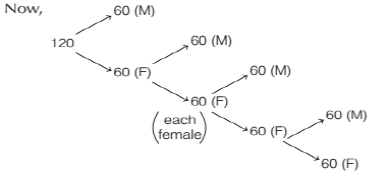Hence, total number of eggs hatched = 60 x 60 x 60 x 60 = 12960000

3. A + B means A is sister of B, A/B means A is  son of B, A = B means A is brother of B, A @ B means A is father of B.
Which of the following shows M is grandson of P?

(a) P = Q/M
(b) P @ B = S @ M
(c) P @ Q + M
(d) P/Q/S + M
Ans. (b)
Solution: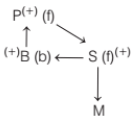From the given options, option (b) only fits for the solution. Although, in this option also it is not confirmed whether M is grandson or granddaughter of P.

4. In a certain code language, if INDIA is written as 95491, then JAPAN is written as
(a) 81815
(b) 11614
(c) 11715
(d) 101614
Ans.
(c)
Solution:
The alphabets from A to I are coded as numerals 1 to 9. The same trend starts from J to R and from S to Z.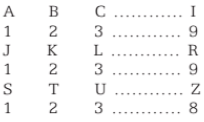Hence, from the above codes,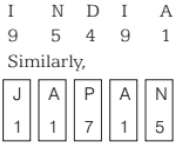5. If 1st June, 2013 is Saturday, then 1st June, 1981 is which of the following?
(a) Monday
(b) Tuesday
(c) Saturday
(d) Thursday
Ans.
(a)
Solution:
From 1st June, 2013 to 1st June, 1981 there are 32 years.
So, there are 32 odd days. The leap years between 1981 to 2013 are 1984, 1988, 1992, 1996, 2000, 2004, 2008, 2012
∴ Total = 8 years
Hence, the total number of odd days = (32 + 8) ÷ 7 = 40 ÷ 7 days = 5 odd days.
As 1st June, 2013 is Saturday. 1st June, 1981 will be 5 days behind Saturday that is Monday.

6.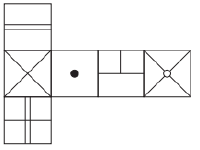An unfolded dice is given above. Find the correct answer which can be formed by folding the unfolded dice.
(a)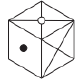(b)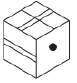(c)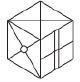(d)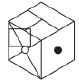Ans. (c)
Solution:
The opposite faces of the dice will be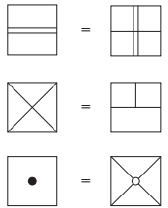Hence, the dice formed will be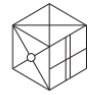7. Fill in the missing block logically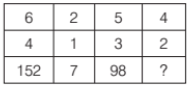(a) 58
(b) 48
(c) 56
(d) 72
Ans. (c)
Solution:
63 – 43 = 216 – 64 = 152
23 – 13 = 8 – 1 = 7
53 – 33 = 125 – 27 = 98
43 – 23 = 64 – 8 = 56

8. Anurag’s grandfather has an old cuckoo clock. It takes 5 s for the cuckoo clock to chime 5 cuckoos. How long will it take to chime 10 cuckoos?
(a) 10 s
(b) 11 s
(c) 10: 25 s
(d) 11: 25 s
Ans.
(d)
Solution:
It takes 5 s for the cuckoo clock to chime 5  cuckoos. i.e. 1st cuckoo clock chimes at t = 0 s
5th cuckoo clock chimes at t = 5 s
Time interval = 5/4 s = 1.25 s
For the 9th chime = × = 9 x 1.25 = 11.25 seconds
Hence, the time taken to chime 10 cuckoos is  11: 25 s.

9. Deepesh wants a house such that all sides of the house face North he should build the house ……
(a) on the South pole
(b) on the North pole
(c) at the equator
(d) at an angle of 120° with the East direction
Ans. (a)
Solution:
The house build at South pole has all its sides facing North. And the house build at North pole has all its sides facing South.

10. In a shouting game, Rohit and Shiv responded in the proportion of Ram and Shyam’s shouting.
Study the table and answer accordingly, what will come at the place of question mark(?)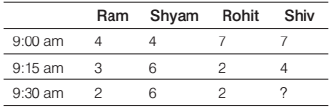(a) 4
(b) 8
(c) 6
(d) 2
Ans. (c)
Solution:
At 9:00 am the ratio of shouting of Ram and Shyam is 1: 1, hence the responding of Rohit and Shiv is at a ratio of 1: 1. Similarly,  At 9: 15 am the ratio is 1: 2.
At 9: 30 am the ratio is 1: 3.
Hence, 1/3 = 2/x ⇒ x = 6

11. A statement is followed by two Courses of action I and II. Assume everything in the statement to be true. Decide which of the two given suggested courses of action logically follows for pursuing. Statement Since 2018, the bulk of India’s population has comprised of young working people, much more than the dependent population, children below 5 years of age and old people above 65 years of age. This trend will continue for the next 55 years.
Courses of Action
I. There will be a huge increase in the GDP of the country.
II. According to a report by UNFPA, this population will be able to contribute effectively, if good health facilities, education and proper infrastructure are provided to the whole population.

(a) None follows
(b) Only I follows
(c) Only II follows
(d) Both I and II follow
Ans.
(c)
Solution:
Only II logically follows, as if good health facilities, education and proper infrastructure are provided to the whole population, the population will be of working people for the next coming years.

12. A clock gains 10 min a day. The clock was  corrected at 6:00 am. what will be the correct  time when the clock shows 11:00 am, following  day?
(a) 10: 48 am
(b) 11: 00 am
(c) 11: 45 am
(d) 10: 30 am
Ans. (a)
Solution:
Normal clock - in 24 h = 1440 min
Defective clock - in 24 h = 1450 min
From today’s 6 am to the following day 11:00 am. So, it will be 29 hours.
For the defective clock the minutes will be  29 60 1740  × = min Now, following the ratio pattern,
Normal clock – 1440      ?
Defective clock – 1450   1740
29 x 50   29 x 60
Ratio = 5 : 6
Making the equation:
5/6 = 1440/x
x = 1728 mins
1728 min = 28 h 48 min which will be 10:48 am.

13. Saddam takes 3 pills with a gap of 30 min. What is the minimum time by which Saddam will get rid of his pain?
(a) 90 min
(b) 60 min
(c) 58 min
(d) 100 min
Ans.
(b)
Solution:
Let the time at which pill is taken be ‘t’. So, first pill taken at t1 = 0 min, 2nd pill taken at t2 = 30 min  and 3rd pill taken at t3 = 60 min  Hence, after 60 min Saddam will get rid of his pain.

14. In a mobile manufacturing company 6 staff members packed 6 mobiles in 6 min. The management wants 60 mobile to be packed in 60 min. How many staff member in total are required?
(a) 60
(b) 360
(c) 600
(d) 6
Ans.
(d)
Solution:
M1T1/W1 = M2T2/W2
where, M = Number of men
T = Time taken for doing the work,
W = Work done
M1 = 6       M2 = k
T1 = 6 min T2 = 60 min
W1 = 6 mobiles W2 = 60 mobiles
∴ (6 x 6)/6 = (k x 60)/60
k = 6 staff members
Hence, 6 staff members in total are required to pack 60 mobiles in 60 min.

15. Choose the word that will complete the second pair in the same way as first pair.
Ornithologist: Bird:: Herpetologist:
(a) Snakes
(b) Reptiles
(c) Mammals

(d) None of these
Ans. (b)
Solution:
As ornithologist studies birds, similarly herpetologist studies reptiles.

16. Amar consumed 100 Sweets from Monday to Friday. Each day, he consumed 6 more Sweets than the previous day. How many Sweets did he consume on Wednesday?
(a) 12 Sweets
(b) 20 Sweets
(c) 24 Sweets
(d) 8 Sweets
Ans.
(b)
Solution:
The total Sweets consumed in the 5 days from Monday to Friday = 100
Let Sweets consumed on Monday = a
There is an increase of 6 Sweets in consumption from the previous day.
Therefore,
a + (a + 6) + (a + 12) + (a + 12) + (a + 18) + (a + 24) = 100
5a + 60 = 100
Sweets consumed on Monday is 8.
Sweets consumed on Wednesday =  8 + 12 = 20 Sweets

17. Find the missing term in the following series 0, 1, 2, 5, 20, 25,?,
157,
(a) 35
(b) 150

(c) 145
(d) 40
Ans.
(b)
Solution:
There is a pattern which is as follows:
0 + 1 = 1
1 x 2 = 2
2 + 3 = 5
5 x 4 = 20
20 + 5 = 25
25 x 6 = 150
Hence, option (b) is the answer.

18. Which of the answer figures given will complete the pattern of the question figure?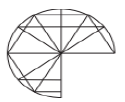(a)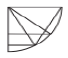(b)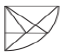(c)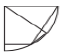(d)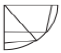Ans.
(a)
Solution:
Clearly, option figure (a) completes the original figure which looks like the figure as shown below.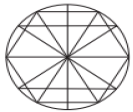19. Find what will come at the place of question mark(?).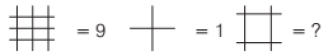(a) 4
(b) 8
(c) 6
(d) 16
Ans. (a)
Solution:
The intersection points in the figure are taken as the answer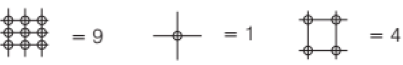20. Rajnath says, “that man’s father is my father’s  son”. How is that man related to Rajnath?
(a) Uncle
(b) Son
(c) Rajnath himself
(d) Cousin
Ans.
(b)
Solution:
Rajnath’s father’s son will be either Rajnath himself or his brother. So, the man will be either Rajnath’s  son or his nephew. From the given options, the man will be Rajnath’s son.

21. How many times does the letter ‘A’ appear from 0 to 100?
(a) 2
(b) 5
(c) 1

(d) None of these
Ans. (d)
Solution:
The letter ‘A’ appears first time in the word  ‘thousand’. So, the answer will be (zero) ‘0’ which is not given  in the options. So, option (d) will be the answer.

22. Population of Honolulu (2 years ago) was 125000. Due to natural calamities people started migrating. So, population decreased at  the rate of 4% per annum. How many migrated  from this town in past 2 years?
(a) 115200
(b) 105000
(c) 9800

(d) None of these
Ans. (c)
Solution:
The population 2 yr ago = 125000
Decrease in population = 4% per annum
The present population of the town
(96/100) x (96/100) x 125000 = 115200
People migrated from the town in past 2 years = 125000 – 115200 = 9800

Directions (Q. Nos. 23-26) Read the given information carefully and answer the questions given below.
Five movies – Do Bigha Jameen, Sholay, 3 idiots, Chak De, Aanand screening on Monday to Friday in any order. Movie screened on Friday remains till Sunday. Screening of Do Bigha Jameen and Chak De should not  be on first and last day. Chak De should be followed by Aanand. Sholay is screened immediately after Do Bigha Jameen. There is only one movie screened between Sholay and 3 idiots.
23. Which movie was screened on Friday?
(a) Aanand
(b) Chak De
(c) 3 Idiots
(d) Do Bigha Jameen
Ans:
(a)
Solution:
There is a chart of movies with their respective days:
Mon - 3 idiots
Tue - Do Bigha Jameen
Wed - Sholay
Thurs - Chak De
Fri - Aanand
The movie Aanand was screened on Friday.

24. Sholay was screened on which day?
(a) Monday
(b) Friday
(c) Wednesday
(d) None of these
Ans.
(c)
Solution:
There is a chart of movies with their respective days:
Mon - 3 idiots
Tue - Do Bigha Jameen
Wed - Sholay
Thurs - Chak De
Fri - Aanand
The movie Sholay was screened on Wednesday.

25. 3 idiots was screened on which day?
(a) Friday

(b) Wednesday
(c) Tuesday
(d) Monday

Ans. (d)
Solution:
There is a chart of movies with their respective days:
Mon - 3 idiots
Tue - Do Bigha Jameen
Wed - Sholay
Thurs - Chak De
Fri - Aanand
The movie 3 idiots was screened on Monday.

26. Rahul asked Shyam to find N such that N! > 10N and must be the smallest integer. Shyam calculates and find N is between 10 to 15, but he  asks his friend Sohan who says it is between 16  to 20. Then, Shyam asks his neighbour Suresh who says it is between 21 to 25. Then, he asks  his cousin Sonal who says it is 15 between 26 to  31. Who is correct?
(a) Suresh
(b) Sonal
(c) Shyam
(d) None of them
Ans. (a)
Solution:
From 1, 2, 3, 4 …………… 19, 20
it is not possible i.e N! is not greater than 10N
For 25! > 1025 It is possible.
Hence, Suresh was correct.

Directions (Q. Nos. 27-31) Read the given information  carefully and answer the questions given below.
Eight boxes namely H to O are placed in a rack one  above the other. The lowermost rack is numbered as 1 and above is 2 and so on. Each of the boxes is having different colors among Blue, Green, Yellow, Red, White, Orange, Indigo and Brown. Each of the boxes is having  different serial numbers among 19, 23, 17, 31, 34, 37, 27  and 21. All the above information is not necessarily in the same order.
There are three boxes placed between the box which  having serial number as 27 and the Indigo colored box. Box J’s serial number is not 27. The Indigo colored box is placed adjacent to blue colored box. Box I’s serial  number is 37 and is not placed at the topmost rack. Box M is yellow colored and placed adjacent to the Red colored box. Box H is placed in the even numbered rack above fourth and it is placed adjacent to box which has serial number as 31. Not more than five boxes are placed above the Red colored box. Box J is placed in the seventh rack and Box H is placed adjacent to Box J. Two boxes are placed between the boxes having the serial number 21 and 34. Box J is White colored. Maximum numbers of boxes are placed between the Brown colored box and Orange colored box. Box H is not Brown colored. Box L is Green colored and it is placed adjacent to the Orange colored box. Box N’s serial number is 21 and placed in the lowermost rack. The box which has serial number as 23 is placed three boxes above the Yellow colored box.
Box O’s serial number is 19. The box which has serial  number as 17 is placed above the box which has serial number as 34.
27. What is the serial number of Box H?
(a) 23
(b) 33
(c) 17
(d) 27

Ans. (c)
Solution:
There is a table which includes every information given in the set, which is as follows: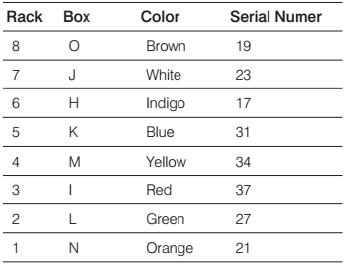The serial number of Box H is 17.

28. Which among the following combination is true?
(a) Box N - Brown - 21 - 1st rack
(b) Box M - Yellow - 34 - 3rd rack
(c) Box K - Blue - 31 - 5th rack
(d) Box O - Orange - 19 - 8th rack
Ans. (c)
Solution:

There is a table which includes every information given in the set, which is as follows:Box K - Blue - 31 - 5th rack is the correct  combination.

29. In certain way Box O is related to serial number 17, Box H is related to serial number 34 and in same way Box M is related to which of the following serial number?
(a) 21
(b) 23
(c) 37
(d) 27

Ans. (d)
Solution:
There is a table which includes every information given in the set, which is as follows:As, Box O → −2 Serial number 17  and Box H → −2 Serial number 34  Similarly, Box M → −2 Serial number 27;  Box M is related to serial number 27.

30. Which of the following statement is true?
(a) Only two boxes are placed below Box M
(b) Box H is placed two boxes above the Red colored box
(c) Box L and Box K are placed adjacent to the box which has serial number 34
(d) None of the above
Ans. (d)
Solution:
There is a table which includes every information given in the set, which is as follows:None of the given option is true. Hence, the  correct answer is option (d).

31. Three of the following four are alike in a certain way and hence form a group. Which of the following does not belong to the group?
(a) Box L - 34
(b) Box K - 23
(c) Box H - 19
(d) Box O - 17
Ans. (b)
Solution:
There is a table which includes every information given in the set, which is as follows:Following the common explanations, Box K is placed in 5th rack and Box J (serial number 23) is  placed in 7th rack i.e. odd number combination. Hence, option (b) does not belong to the group.

Directions (Q. Nos. 32 and 33) In each group of question below are two conclusions followed by five set of  statements. You have to choose the correct set of statements that logically satisfies given conclusions.
32. Conclusions
At least some states are district.
Some towns being district is a possibility.
Statements
I. All districts are states. Some states are village. No town is district.
II. No districts is states. Some states are towns. No town is village.
III. All districts are states. Some states are towns. No town is village.
IV. No districts is states. Some states are towns. No town is village.
V. All districts are states. Some states are village. Some town are village.
(a) Only Statement I
(b) Both Statements II and III
(c) Only Statement VI
(d) Both Statements III and V
Ans.
(d)
Solution: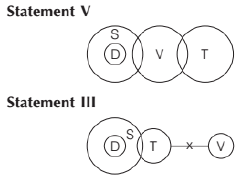Hence, both Statements III and V follow.

33. Conclusions
No car is a bike.
All bikes being vans is a possibility.
Statements
I. All scooters are bikes. No bike is a moped. All cars are bikes. All mopeds are vans
II. Some scooters are bikes. No bike is a van. All cars are mopeds. All mopeds are vans.
III. Some scooters are bikes. No bike is a  moped. All cars are bikes. All mopeds are vans.
IV. All scooters are bikes. No bike is a van. All cars are mopeds. All mopeds are vans.
V. All scooters are bikes. No bike is a moped. All cars are mopeds. All mopeds are vans
(a) Only Statement V
(b) Only Statement I
(c) Only Statement III
(d) Both Statements III and IV

Ans. (a)
Solution: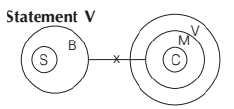Hence, only Statement V follows.

34. In a coded language LAUGH is written as 62 7 107 37 42, then SMILE will be written as (Note Do not include spaces in your answer.)
(a) 97 67 47 62 27
(b) 67 42 47 62 27
(c) 97 65 45 62 28
(d) 97 67 47 61 27

Ans. (a)
Solution:
LAUGH is written as 62 7 107 37 42 In the alphabetical series order.
L = 12 A = 1 U = 21 G = 7 H = 8
The pattern in this question is:
(n x 5) + 2
62 = 12 x 5 + 2
7 = 1 x 5 + 2
107 = 21 x 5 + 2
37 = 7 x 5 + 2
42 = 8 x 5 + 2
Similarly, S M I L E
S =19 M = 13  I = 9 L = 12 E = 5
19 x 5 + 2 = 97
13 x 5 + 2 = 67
9 x 5 + 2 = 47
12 x 5 + 2 = 62
5 x 5 + 2 = 27
Hence, the code for SMILE will be  97 67 47 62 27

35. If ‘+’ means ‘×’, ‘−’ means ‘÷’, ‘÷’ means ‘+’ and ‘×’  means ‘−’, then what will be the value of  16 ÷ 64 – 4 x 4 + 3 = ?
(a) 20
(b) 52
(c) 15
(d) 12
Sol.
(a)
Solution:
16 ÷ 64 – 4 x 4 + 3 = ?
16 + 64 ÷ 4 – 4 × 3  = ?
16 + 16 − 4 × 3 = ?
32 − 12  = ?
? = 20

36. The question below is followed by two Arguments I and II. You have to decide which of the argument is a ‘strong’ argument and which is a ‘weak’ argument? Statement Should young entrepreneurs be encouraged? Arguments I. Yes, they will help in the industrial development of the country. II. Yes, they will reduce the burden on the employment market.
(a) If only Argument I is strong
(b) If only Argument II is strong
(c) If both I and II are strong
(d) If either I or II is strong
Ans.
(c)
Solution:
It is very clear that encouragement of the young entrepreneur will open up the fields for setting up of new industries. Therefore, it will help in industrial development. Consequently, more job opportunities will be created. Thus, both the arguments are strong.

The document Practice Doc: SNAP 2019 For Analytical & Logical Reasoning Notes - CAT is a part of CAT category.
All you need of CAT at this link: CATUse Code STAYHOME200 and get INR 200 additional OFF

### How to Prepare for CAT

Read our guide to prepare for CAT which is created by Toppers & the best Teachers

Track your progress, build streaks, highlight & save important lessons and more!

,

,

,

,

,

,

,

,

,

,

,

,

,

,

,

,

,

,

,

,

,

;